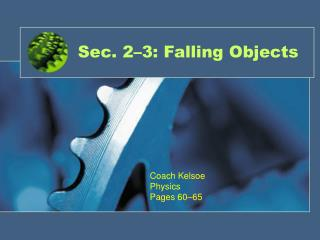DownloadDownload PresentationSec. 2–3: Falling Objects

# Sec. 2–3: Falling Objects

Télécharger la présentation## Sec. 2–3: Falling Objects

- - - - - - - - - - - - - - - - - - - - - - - - - - - E N D - - - - - - - - - - - - - - - - - - - - - - - - - - -
##### Presentation Transcript

1. Sec. 2–3: Falling Objects Coach Kelsoe Physics Pages 60–65

2. Objectives • Relate the motion of a freely falling body to motion with constant acceleration. • Calculate displacement, velocity, and time at various points in the motion of a freely falling object. • Compare the motions of different objects in free fall.

3. Free Fall • Free fall is the motion of a body when only the force due to gravity is acting on the body. • The acceleration on an object in free fall is called the acceleration due to gravity, or free-fall acceleration. • Free-fall acceleration is denoted with the symbols ag (generally) or g (on Earth’s surface).

4. Free-Fall Acceleration • Free-fall acceleration is the same for all objects, regardless of mass. • Our book will use the value g = -9.81 m/s2. • Free-fall acceleration on Earth’s surface is -9.81 m/s2 at all points in the object’s motion. • Consider a ball thrown up in the air. • Moving upward: the velocity is decreasing, acceleration is -9.81 m/s2 • Top of path: velocity is zero, acceleration is -9.81 m/s2 • Moving downward: velocity is increasing, acceleration is -9.81 m/s2.

5. Sample Problem Falling Object Jason hits a volleyball so that it moves with an initial velocity of 6.0 m/s straight upward. If the volleyball starts from 2.0 m above the floor, how long will it be in the air before it strikes the floor?

6. Sample Problem Solution • Define • Given: • vi = +6.0 m/s • a = -g = -9.81 m/s2 • Δy = -2.0 m • Unknown: • Δt = ? • Diagram: • Place the origin at the starting point of the ball (yi = 0 at ti = 0).

7. Sample Problem Solution • Plan • Choose an equation or situation: • Both ∆t and vf are unknown. Therefore, first solve for vf using the equation that does not require time. Then, the equation for vf that does involve time can be used to solve for ∆t. • vf2 = vi2 + 2aΔy vf = vi + aΔt • Rearrange the equation to isolate the unknown. • Take the square root of the first equation to isolate vf. The second equation must be rearranged to solve for ∆t. • vf = ±√vi2 + 2aΔy Δt = vf – vi/a

8. Sample Problem Solution • Calculate • Substitute the values into the equation and solve: • First find the velocity of the ball at the moment that it hits the floor. • vf = ±√vi2 + 2aΔy • vf = ±√(6.0 m/s)2 + 2(-9.81 m/s2)(-2.0 m) • vf = ±√36 m2/s2 + 39 m2/s2 = -8.7 m/s • Tip: When you take the square root to find vf , select thenegative answer.

9. Vocabulary